### SUMMARY: A GENERAL METHODOLOGY FOR LINEAR LEAST SQUARES

To repeat myself, the examples given above are all called "linear least squares." They are "least squares" because we derive our answers by minimizing the weighted sum of the squares of the residuals,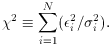They are called "linear" because the equations which we must solve are linear in the unknown quantities a, b, c, . . ., and not because we are fitting a "straight line" to the data. As I have already stated, these methods may easily be generalized to larger problems.

Notation.

We have N sets of observations, each observation consisting of values for n variables tj, i (j = 1, . . . , n) which are known perfectly, and one dependent variable yi, which is subject to observational error. Thus, we have N relations of the form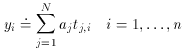involving the n unknown quantities, or fitting parameters, aj.

Problems 1 and 2:     y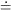mx + b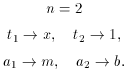Problem 3:     yax2 + bx + cProblem 4:     ra1 cos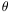+ a2 cos+ a3 cossin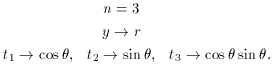Step 1.

Write the equation in the formStep 2.

Form the n x n matrix M and the n x 1 column vector V: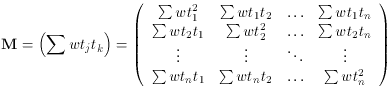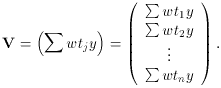Remember, wi = s2 /i2. If1 =2 = . . .N =, you might as well set s =and give every observation weight 1.

Step 3.

Compute the n x 1 column vector containing the best estimates of the fitting parameters A :Step 4.

Compute the mean error of unit weight: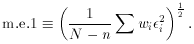If you thought you knew your errors, but the m.e.1 comes out grossly different from whatever value of s you adopted, then you've got a problem. Most likely you've mis-estimated your errors, or maybe your model is just wrong. (Remember that it's usually simplest to use s1; then, you expect m.e.11 to come out at the end.) If you didn't know your errors to begin with, or rather if you only knew them to within a scaling factor, m.e.1 is your best guess at that scaling factor.

I guess that's pretty much it for the elegant theory behind linear least squares. If you know enough computer programming to set up a matrix, and if you have access to a math library with a matrix inverter, YOU TOO can now do linear least squares to solve all sorts of problems. (Of course, if you don't like to use computers, you can always do it by hand!)

Now I'd like to show you a few things which will allow you to do least squares just like the pros - a few tricks that you might not have thought of.